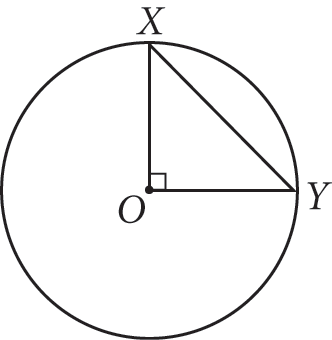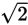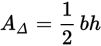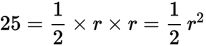# SAT Math Multiple Choice Question 973: Answer and Explanation

### Test Information

Question: 973

9.In the figure above, O is the center of the circle. If the area of ΔXOY is 25, what is the area of the circle?

• A. 25π
• B. 25π• C. 50π
• D. 625π

Explanation:

C

Difficulty: Medium

Category: Additional Topics in Math / Geometry

Strategic Advice: The two legs of ΔXOY are both radii of the circle and therefore have the same length. Use this fact to find a link between the formulas used to find the area of a triangle and the area of a circle.

Getting to the Answer: Let r represent the length of each leg of ΔXOY. Because the area of a triangle is given byand the area of ΔXOY is 25, it follows that. Therefore, r2 = 50. Substitute this value for r2 into the formula for finding the area of a circle: A° = πr2 = π × 50 = 50π Choice (C) is therefore the correct answer.

(Note that you did not have to find the length of the circle's radius to answer this question; doing so would have cost you extra time.)